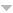# Publications

Found 169 results
[ Author] Title Type Year
Filters: First Letter Of Last Name is Y  [Clear All Filters]
Y
An analog of Morgan's theorem for the Kontorovich-Lebedev transform. Adv. Pure Appl. Math.. 2010;1:159-162.Edit
Certain isometries related to the bilateral Laplace transform. Math. Model. Anal.. 2006;11:331-346.
On the construction of integral transformations by the composition method. Izv. Vyssh. Uchebn. Zaved. Mat.. 1993:71-79 (1994).
On the theory of convolution integral equations related to Lebedev's type operators. Sarajevo J. Math.. 2009;5(17):119-132.
On the least values of \$L_p\$-norms for the Kontorovich-Lebedev transform and its convolution. J. Approx. Theory. 2004;131:231-242.
The generalizations of integral analog of the Leibniz rule on the \$G\$-convolutions. Extracta Math.. 1991;6:119-122.Edit
On the half-Hartley transform, its iteration and compositions with Fourier transforms. J. Integral Equations Appl. . 2014;26(4):581-608.
Index transforms associated with Bessel and Lommel functions. Z. Anal. Anwendungen. 2002;21:627-638.
On the Mehler-Fock transform in \$L_p\$-space. Math. Nachr.. 1997;185:261-277.Edit
On some Rajchman measures and equivalent Salem's problem. Commun. Math. Anal.. 2013;14:28-41.
The Fourier-Stieltjes transform of Minkowski's \$?(x)\$ function and an affirmative answer to Salem's problem. C. R. Math. Acad. Sci. Paris. 2011;349:633-636.
\$L_2\$-interpretation of the Kontorovich-Lebedev integrals. Int. J. Pure Appl. Math.. 2008;42:99-110.
Index transforms with Weber-type kernels . Integral Transforms and Special Functions. 2018;29(3):171-188.
Fundamental solutions of the fractional two-parameter telegraph equation. Integral Transforms Spec. Funct.. 2012;23:509-519.Edit
On the Mehler-Fock index transform in \$L_p\$-space. S\=urikaisekikenky\=usho Kōky\=uroku. 1994:130-144.Edit
Eigenfunctions and fundamental solutions of the fractional two-parameter Laplacian. Int. J. Math. Math. Sci.. 2010:Art. ID 541934, 18.
The Kontorovich-Lebedev transformation on Sobolev type spaces. Sarajevo J. Math.. 2005;1(14):211-234.